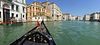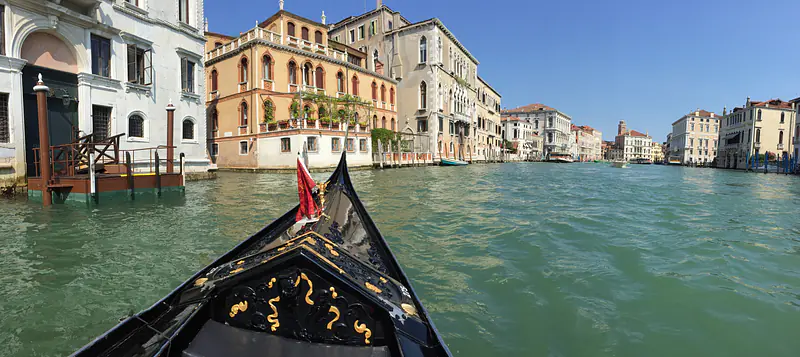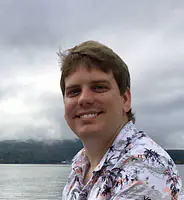# Cody Mischel

PhD Candidate - UIC

Close

# Courses

Fluid mechanics and transport processes involving non-Newtonian fluids. Purely viscous and viscoelastic behavior. Viscometric functions and rheometry. Heat and mass transfer in non-Newtonian fluids.

Continuum theory of momentum, energy, and mass transfer. Viscous behavior of fluids. Laminar and turbulent flow. Thermal conduction and convection, diffusion and coupled operations.

Theoretical and numerical fluid mechanics of microstructure: potential flow and virtual mass, quasistatic versus transient Stokes flow, integral theorems, multipole expansions, singularity solutions, fluctuations, and current applications.

Interfacial phenomena in practice: soap bubbles, emulsions, foams, detergents; surface tension driven flows, Marangoni effect; interfacial rheology; colloids, emulsions, 3D-printing; interfacial thermodynamics, biological interfaces.

Development of concepts and techniques used in mathematical models of fluid motions. Euler and Navier Stokes equations. Vorticity and vortex motion. Waves and instabilities. Viscous fluids and boundary layers. Asymptotic methods.

Advanced mathematical techniques in chemical engineering. Includes infinite series in thermodynamic perturbation theory; Laplace transforms in process control; chemical diffusion transport theories and differential equations.

Advance numerical methods to the solution of complex and non-linear mathematical problems in chemical engineering; includes methods to solve problems arising in phase and chemical reaction equilibria, chemical kinetics, and transport.

Numerical analysis of Finite Difference methods for PDE of mathematical physics: Wave, heat, and Laplace equations. Introduction to numerical analysis of the Finite Element method.

Multiplicities in chemically reacting systems nonideal reactors: Effects of residence time distribution and mixing history. Heterogeneous noncatalytic reactions: gas-liquid, liquid-liquid, and solid-fluid systems.

Laws of thermodynamics. General conditions for equilibrium and stability. Thermodynamic potentials. Phase transition and critical phenomena. Principle of irreversible thermodynamics, Onsager's fundamental theorem and engineering applications.